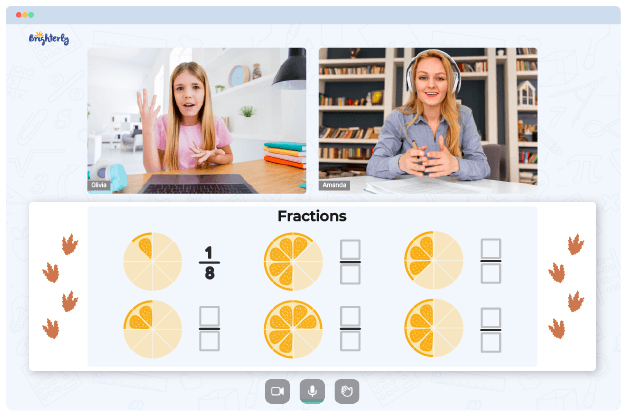# Adding Fractions With Unlike Denominators Worksheets

Students first have a snippet of fractions in elementary and middle school. Generally, this concept helps students learn about halves and unequal numbers in mathematics.

But teachers need to give students time and attention to understand the link between fractions, decimals, and percents and their applications. Any reliable adding fractions with unlike denominators worksheet can help students establish this connection faster.### Adding Fractions With Unlike Denominators PDF

Adding Fractions With Unlike Denominators Worksheet### Adding Fractions With Unlike Denominators PDF

Adding Fractions With Different Denominators Worksheet### Adding Fractions With Unlike Denominators PDF### Adding Fractions With Unlike Denominators PDF

Adding Fractions With Different Denominators Worksheets

Since students follow various methods for adding and subtracting fractions, it is essential to know if fractions have the same or different denominators. This type of fraction differs from the conventional calculation like ½ + ½ = 1. So, when handling problems involving adding and subtracting fractions, students will benefit from adding fractions with different denominators worksheet as it simplifies their work.

## Steps to Take When Working with Add Fractions with Unlike Denominators Worksheet

Math for Kids

Is Your Child Struggling With Math?
1:1 Online Math TutoringIn case you want to add ½ + ⅗; the conventional steps you can use to learn how to add fractions with unlike denominators with worksheets are:

Step 1: Since the denominators of the fractions are different, first, get the least common multiple (LCM) of 2 and 5. The LCM of 2 and 5 is 10.

Step 2: Next, replace 2 and 5 with 10 and make the equation 2/10 + 5/10. So, we now have a common denominator.

Step 3: Divide the denominator by the numerators: 10/2, and 10/5 = 5 + 6/ 10 = 11/10

## Examples of How an Adding Unlike Fractions Worksheet Can Help Students

Once denominators are equal, adding fractions with different denominators is easy. Denominators should be the same for dealing with improper fractions with greater numerators. After that, sum up denominators.

Adding fractions with different denominators worksheets can help students identify and solve fraction problems. Turn mixed integers into improper fractions and make each bit equal if you combine them. Any math problem will be much easier to solve this way.

For instance, worksheets will introduce you to a rectangular model where you can add fractions ⅓ and ½, respectively. Partitioning the model into three sections and shading one area represent the fraction ⅓. Splitting it into two rows and coloring one part distinguish half of a whole.

A worksheet visualizes fractions, a far cry from the abstract math that students learn. Therefore, kids understand basic fraction addition concepts before moving on to more complex modules. Such approach helps them identify and interpret similar divisions in real-life situations like sharing pizza slices.

## Benefits of Adding Fractions with Unlike Denominators Worksheets

Adding fractions with unlike denominators can be a challenging concept for students to grasp. Many students struggle with understanding how to add and subtract fractions when the denominators are different. This is why it’s important to provide worksheets that focus on adding fractions with unlike denominators so that students can properly practice and learn this concept.

These worksheets can be used to help build a stronger foundational understanding of adding unlike fractions, and provide students with the practice needed to master this concept. With these worksheets, students can learn how to add two unlike fractions by finding the least common denominator (LCD), breaking down each fraction into its simplest form or using the distributive property.

In addition, students can learn how to add more than two fractions with different denominators and understand the importance of finding the LCD in order to be successful. They will also gain a better understanding of adding mixed numbers that have unlike denominators by converting them into improper fractions first.

### Fractions Worksheets

Troubles with Fractions?• Does your child struggle to understand fractions lessons?
• Try studying with an online tutor.

Is your child having trouble understanding the concept of fractions? An online tutor could provide the necessary guidance.